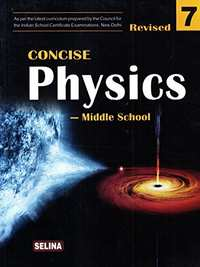ICSE Class 7CISCE
Share

# Selina Solutions for Class 7 Physics

## Solutions for Selina Concise Physics - Middle School for Class 7 (2018-19 Session)

Shaalaa provides Selina Solutions for Class 7 Physics and has all the answers for the questions given in Selina Concise Physics - Middle School for Class 7 (2018-19 Session). Shaalaa is surely a site that most of your classmates are using to perform in exams.

You can solve Selina Concise Physics - Middle School for Class 7 (2018-19 Session) and use Shaalaa Selina Solutions for Class 7 Physics to check your answers.

## Selina Solutions for Class 7 Physics

Given below is chapter wise list for Selina Solutions for Class 7 Physics. Select any chapter number to view solutions.

#### Selina Solutions for Class 7 Physics## Chapters covered in Selina Solutions for Class 7 Physics

#### Chapter 1: Physical Quantities and Measurement

Shaalaa has a total of 66 questions are solved for this Chapter in Class 7 Physics. Chapters covered in Physical Quantities and Measurement are Concept of Physical Quantities and Measurement, Concept of Unit Volume, Estimate the Area of Irregular Shape Using a Graph Paper, Measurement of Area, Measurement of Density of Regular Solids - Formula, Measurement of Densiy of Regular Solids - Basic Concept, Measurement of Volume (3d Concept)

#### Chapter 2: Motion

Shaalaa has a total of 65 questions are solved for this Chapter in Class 7 Physics. Chapters covered in Motion are Concept of Force and Pressure: Motion, Differences Between Mass and Weight, Motion as a Change in Position of an Object with Respect to Time, Types of Motion - Circulatory, Types of Motion - Oscillatory, Types of Motion - Random, Types of Motion - Repetitive (Periodic and Non Periodic), Types of Motion - Translatory, Uniform and Non Uniform Motion: Concept of Distance and Speed (Average Speed), Weight - Concept

#### Chapter 3: Energy

Shaalaa has a total of 63 questions are solved for this Chapter in Class 7 Physics. Chapters covered in Energy are Concept of Law of Conservation of Energy, Different Forms of Energy, Energy as Capacity to Do Work, Inter-conversion of Energy, Law of Conservation of Energy: Real World Examples, Units of Energy (Joule and Calorie)

#### Chapter 4: Light Energy

Shaalaa has a total of 70 questions are solved for this Chapter in Class 7 Physics. Chapters covered in Light Energy are Appearance of Colour of an Object (Based on Reflection and Absorption), Colour Subtraction, Concept of Light Energy, Concept of Reflection, Formation of Secondary Colours by Colour Addition., Laws of Reflection, Plane Mirror - Characteristics of the Image Formed (Lateral Inversion, Same Size, Distance is Preserved)., Plane Mirror - Ray Diagram (No Mention of Virtual Image), Plane Mirror - Uses, Primary Colours (RGB), Speed of Light (3 X 10 8 M/S)., Terms Related to Reflection:Normal, Plane, Point of Incidence, Angle of Incidence, Angle of Reflection

#### Chapter 5: Heat

Shaalaa has a total of 96 questions are solved for this Chapter in Class 7 Physics. Chapters covered in Heat are Concept of Heat, Different Units of Temperature (°C, °F, K)., Effects of Heat - Change in Size (Expansion and Contraction)., Effects of Heat - Change in State, Effects of Heat - Change in Temperature, Effects of Heat - Choice of Conductors and Insulators in Day to Day Life (Pan Handles, Metal Cooking Utensils Etc.), Effects of Heat - Good Conductors and Bad Conductors of Heat and Their Examples, Heat as a Form of Energy and Its Units, Joule(J) and Calorie (Cal)., Methods of Heat Transfer - Conduction, Methods of Heat Transfer - Convection, Methods of Heat Transfer - Radiation

#### Chapter 6: Sound

Shaalaa has a total of 74 questions are solved for this Chapter in Class 7 Physics. Chapters covered in Sound are Characteristics of a Sound Wave: Amplitude (Relate Amplitude with Loudness), Characteristics of a Sound Wave - Frequency, Concept of Sound, Reflection and Absorption of Sound, Relative Speed of Sound in Different Mediums, Sound as a Longitudinal Wave, Sound Needs a Medium to Propagate, Sources of Sound

#### Chapter 7: Electricity and Magnetism

Shaalaa has a total of 68 questions are solved for this Chapter in Class 7 Physics. Chapters covered in Electricity and Magnetism are Battery as a Collection of Cells Connected in Series, Concept of Electricity and Magnetism, Electric Cell as Source of Electricity, Electric Current as a Flow of Charges, Electromagnetism, Electromagnets and Their Applications- Electric Bell, Good and Bad Conductors of Electricity, Laws of Magnetism, Resistors as Components that Oppose the Flow of Current, Simple Electric Circuit - Series and Parallel, Symbolic Representation of Electrical Components (Key,Battery, Bulb, Conducting Wire, Resistor), Test for a Magnet (By Repulsion)

## Selina Solutions for Class 7 Physics

Class 7 Selina solutions answers all the questions given in the Selina textbooks in a step-by-step process. Our Maths tutors have helped us put together this for our Class 7 Students. The solutions on Shaalaa will help you solve all the Selina Class 7 Physics questions without any problems. Every chapter has been broken down systematically for the students, which gives fast learning and easy retention.

Shaalaa provides free Selina solutions for Class 7 Physics. Shaalaa has carefully crafted Selina solutions for Class 7 Physics that can help you understand the concepts and learn how to answer properly in your board exams. You can also share our link for free Class 7 Physics Selina solutions with your classmates.

If you have any doubts while going through our Class 7 Physics Selina solutions, then you can go through our Video Tutorials for Physics. The tutorials should help you better understand the concepts.

S